Function Repository Resource:

# ParametricSurfaceTangentPlane

Compute the tangent plane of a parametric surface

Contributed by: Wolfram Staff (original content by Alfred Gray)
 ResourceFunction["ParametricSurfaceTangentPlane"][s,{u,v},{u0,v0}] computes the tangent plane at the point (u0,v0) of surface s parametrized by variables u and v.

## Details and Options

ResourceFunction["ParametricSurfaceTangentPlane"] gives an InfinitePlane object.
The equation for the tangent plane of a two-variable function at a particular point can be written as T(x,y)=f(x0,y0)+fx(x0,y0)(x-x0)+fy(x0,y0)(y-y0).
The plane is spanned by two independent vectors normal to the surface normal.
Tangent planes to a surface are planes that touch the surface locally at one point. Globally, more complex intersections may exist.

## Examples

### Basic Examples (2)

Definition of a sphere:

 In:=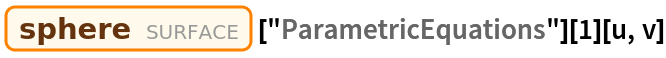Out=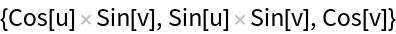Tangent plane of the sphere:

 In:=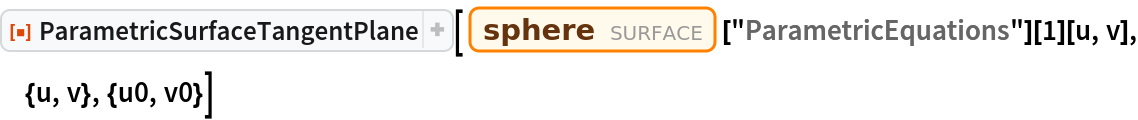Out=Plot the tangent plane at any point on a sphere:

 In:=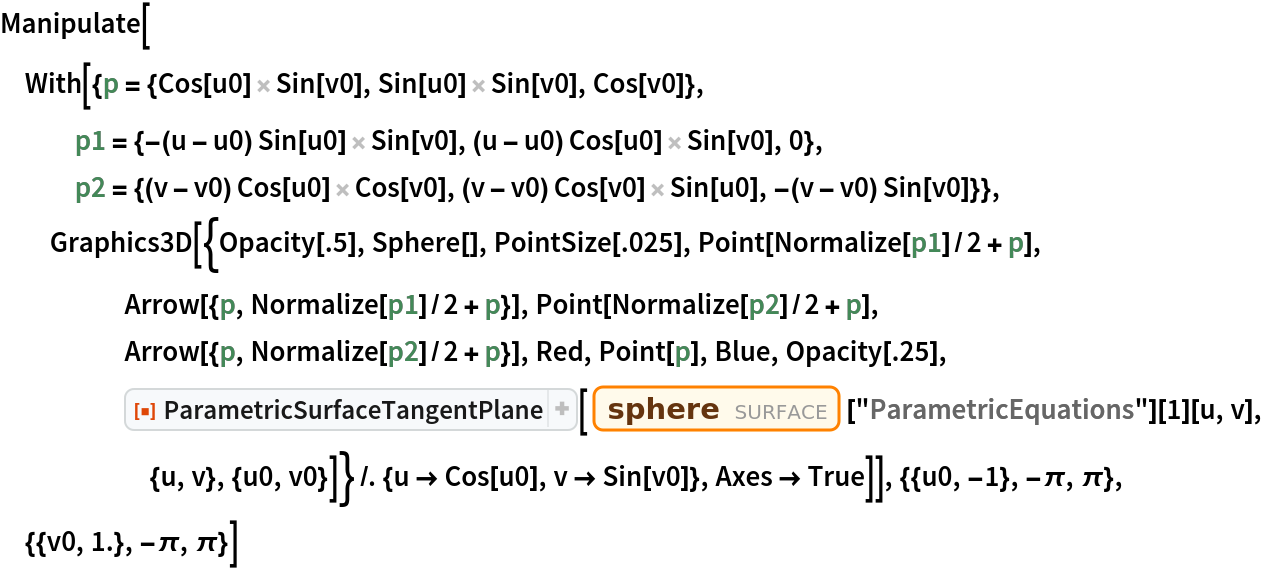Out=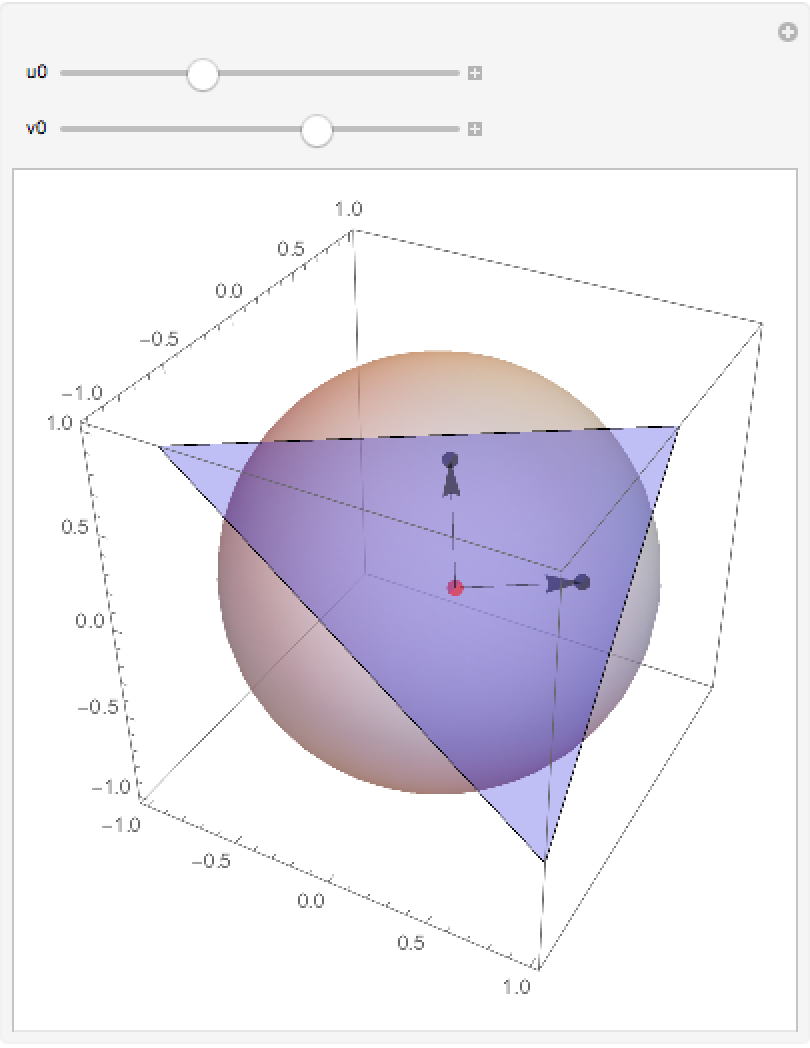Choose a surface:

 In:=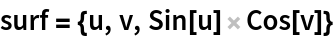Out=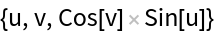Plot it:

 In:=Out=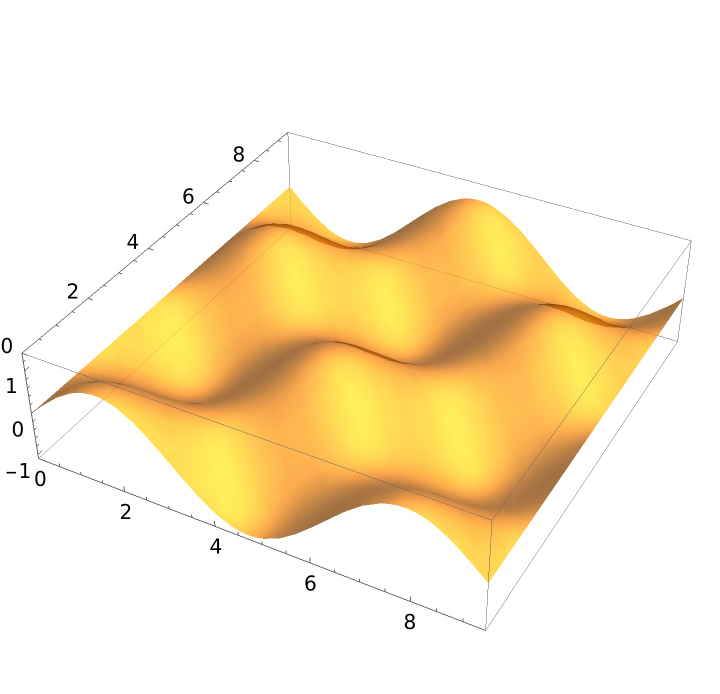Compute the tangent plane of the surface:

 In:=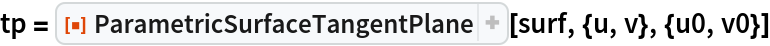Out=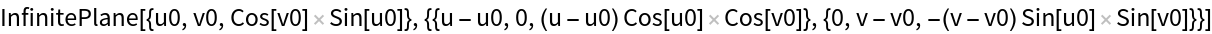Plot the tangent plane at any point (in blue) on the surface; the red curves are the intersection of the plane and the surface:

 In:=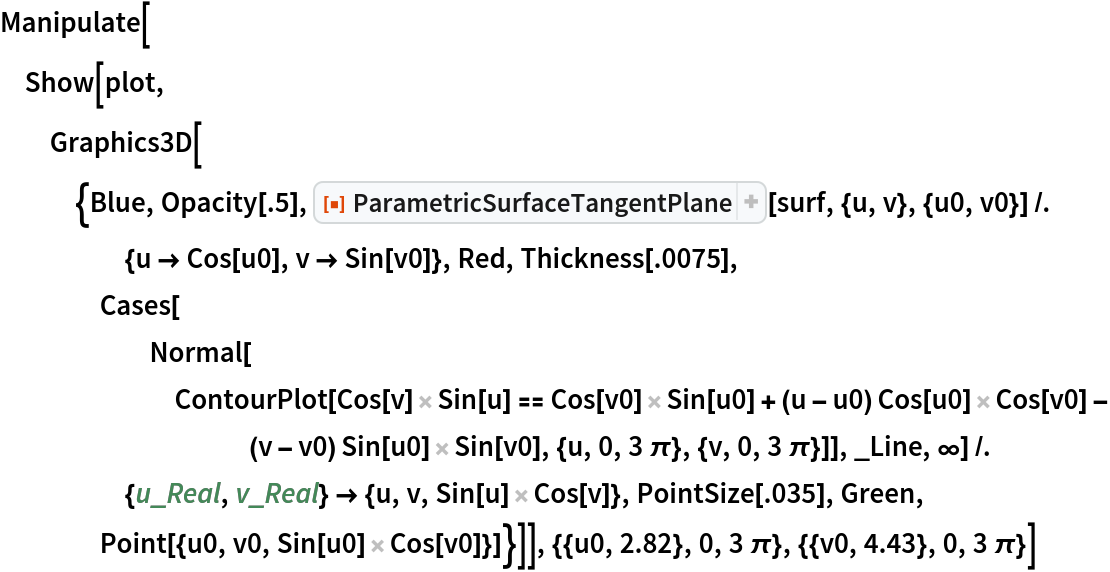Out=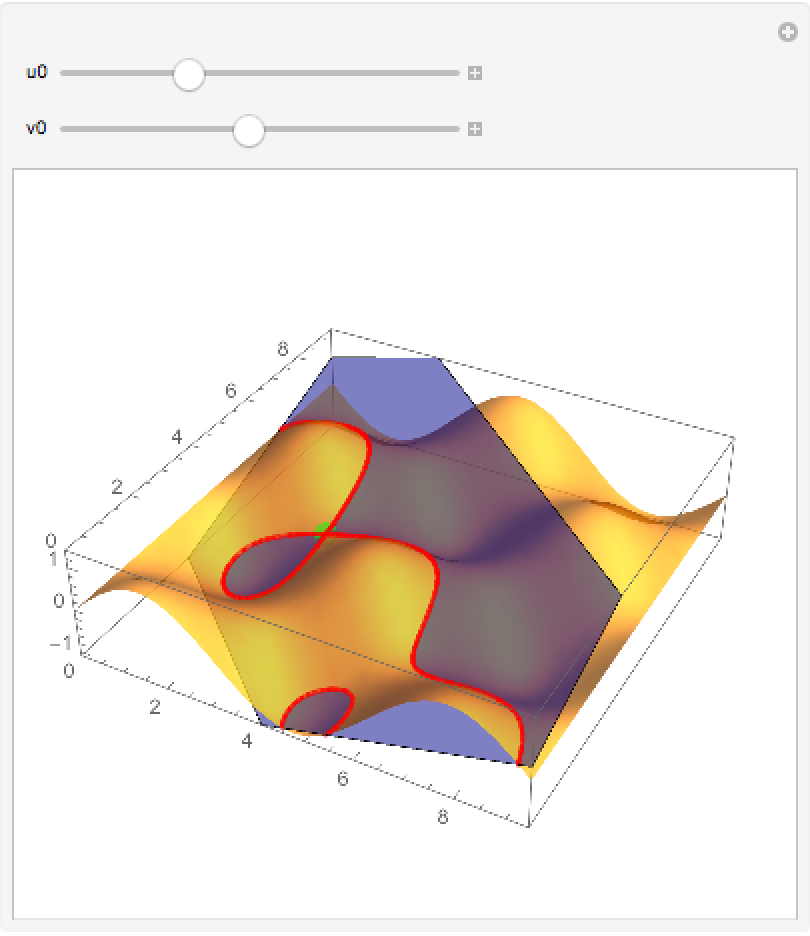Use ClipPlanes to view the surface only at one side of the plane:

 In:=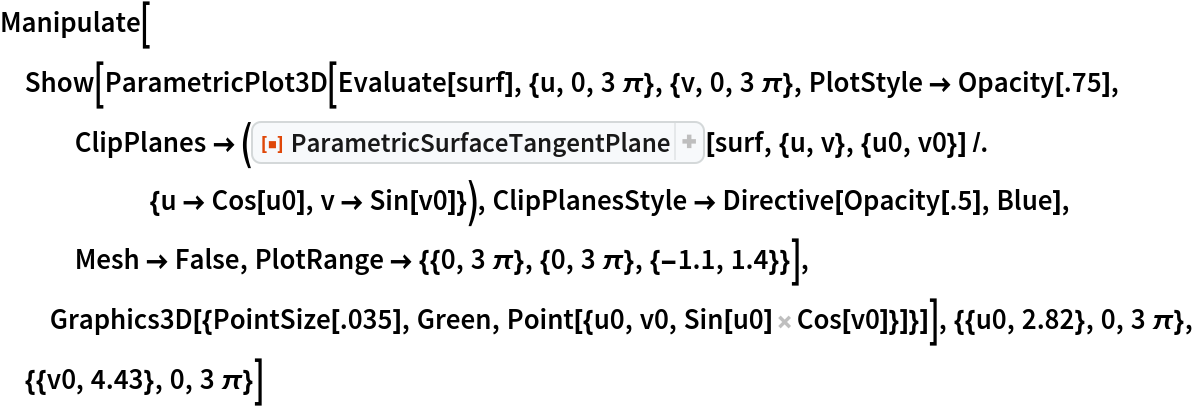Out=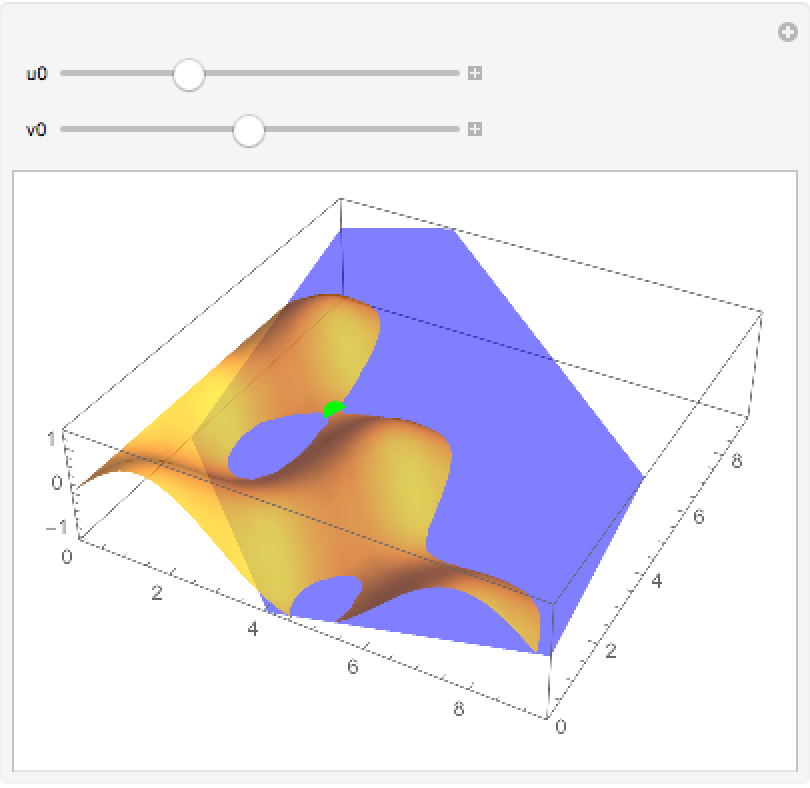Enrique Zeleny

## Version History

• 1.0.0 – 16 July 2020